# Concatenate multiIndex into single index in Pandas Series

• Last Updated : 14 Sep, 2021

In this article, we will see how to concatenate multi-index to a single index in Pandas Series. Multi-index refers to having more than one index with the same name.

Create a sample series:

## Python3

 `# importing pandas module``import` `pandas as pd``import` `numpy as np` `# Creating series data for address details``index_values ``=` `pd.Series([(``'sravan'``, ``'address1'``),``                          ``(``'sravan'``, ``'address2'``),``                          ``(``'sudheer'``, ``'address1'``),``                          ``(``'sudheer'``, ``'address2'``)])` `# assigning values with integers``data ``=` `pd.Series(np.arange(``1``, ``5``),``                 ``index``=``index_values)` `# display data``print``(data)`

Output: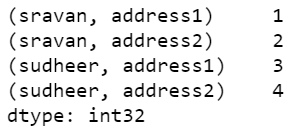Joining two or more data is known as concatenation. Here we are going to concatenate the index using map function.

Syntax:

map(fun, iter)

• fun: function
• iter: iterations.

Below are various examples that depict how to concatenate multi-index into a single index in Series:

Example 1:

This code explains the joining of addresses into one based on multi-index.

## Python3

 `# importing pandas module``import` `pandas as pd` `# Creating series data for address details``index_values ``=` `pd.Series([(``'sravan'``, ``'address1'``),``                          ``(``'sravan'``, ``'address2'``),``                          ``(``'sudheer'``, ``'address1'``),``                          ``(``'sudheer'``, ``'address2'``)])` `# assigning values with integers``data ``=` `pd.Series(np.arange(``1``, ``5``), index``=``index_values)` `# display data``print``(data)` `# mapping with data using '_' symbol with join``data1 ``=` `data.index.``map``(``'_'``.join)` `print``(data1)`

Output: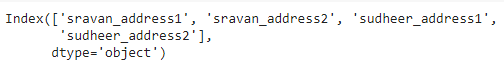Example 2:

This code is an example for all the given same name, but different values passed in a tuple.

## Python3

 `# importing pandas module``import` `pandas as pd` `# importing numpy module``import` `numpy as np` `# Creating series data for address details with same name.``index_values ``=` `pd.Series([(``'sravan'``, ``'address1'``),``                          ``(``'sravan'``, ``'address2'``),``                          ``(``'sravan'``, ``'address3'``),``                          ``(``'sravan'``, ``'address4'``)])` `# assigning values with integers``data ``=` `pd.Series(np.arange(``1``, ``5``),``                 ``index``=``index_values)` `# display data``print``(data)` `# mapping with data using '_' symbol with join``data1 ``=` `data.index.``map``(``'_'``.join)` `print``(data1)`

Output: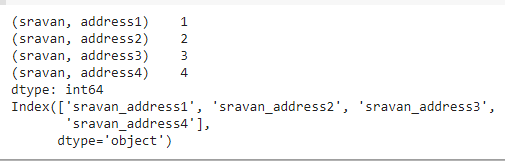Example 3:

This code gives a demo on multiple users given in nested list data structure.

## Python3

 `# importing pandas module``import` `pandas as pd` `# importing numpy module``import` `numpy as np` `# Creating series data for address details``# with same name with nested lists.``index_values ``=` `pd.Series([[``'sravan'``, ``'address1'``],``                          ``[``'sravan'``, ``'address2'``],``                          ``[``'sravan'``, ``'address3'``],``                          ``[``'sravan'``, ``'address4'``],``                          ``[``'vani'``, ``'address5'``],``                          ``[``'vani'``, ``'address6'``],``                          ``[``'vani'``, ``'address7'``],``                          ``[``'vani'``, ``'address8'``]])` `# assigning values with integers``data ``=` `pd.Series(np.arange(``1``, ``9``),``                 ``index``=``index_values)` `# display data``print``(data)` `# mapping with data using '_' symbol with join``data1 ``=` `data.index.``map``(``'_'``.join)` `print``(data1)`

Output: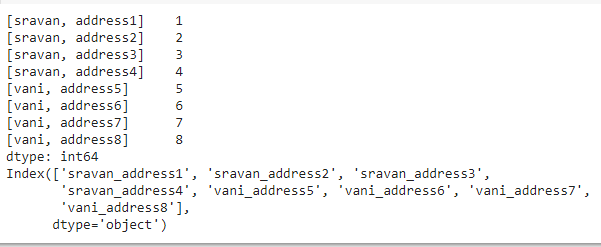Example 4:

This code explains the college data with respect to address passed in a nested list separated by ‘/’ operator.

## Python3

 `# importing pandas module``import` `pandas as pd` `# importing numpy module``import` `numpy as np` `# Creating series data for address details w.r.t``# college names  with same name with nested lists.``index_values ``=` `pd.Series([[``'sravan'``, ``'address1'``, ``'vignan'``],``                          ``[``'sravan'``, ``'address2'``, ``'vignan'``],``                          ``[``'sravan'``, ``'address3'``, ``'vignan'``],``                          ``[``'sravan'``, ``'address4'``, ``'vignan'``],``                          ``[``'vani'``, ``'address5'``, ``'vignan lara'``],``                          ``[``'vani'``, ``'address6'``, ``'vignan lara'``],``                          ``[``'vani'``, ``'address7'``, ``'vignan lara'``],``                          ``[``'vani'``, ``'address8'``, ``'vignan lara'``]])` `# assigning values with integers``data ``=` `pd.Series(np.arange(``1``, ``9``),``                 ``index``=``index_values)` `# display data``print``(data)` `# mapping with data using '/' symbol with join``data1 ``=` `data.index.``map``(``'/'``.join)` `print``(data1)`

Output: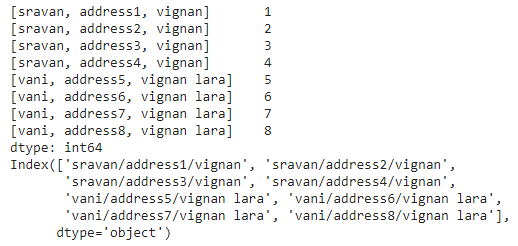My Personal Notes arrow_drop_up